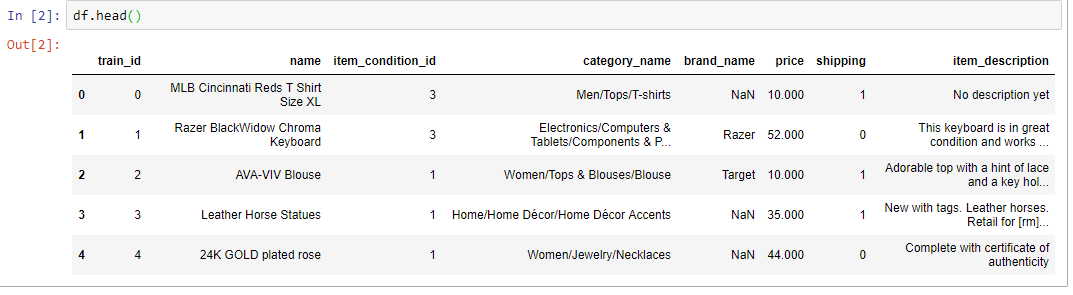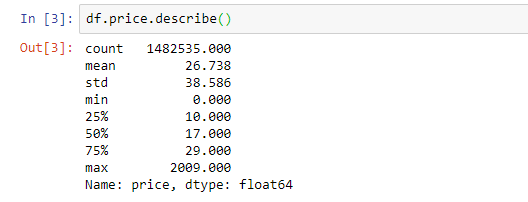## 2019年4月26日 星期五

### [ ML 文章收集 ] ColumnTransformer Meets Natural Language Processing

Source From Here
Preface
How to combine several feature extraction mechanisms or transformations into a single transformer in a scikit-learn pipeline

There are several different methods to append or combine different feature types. One method is using scipy.sparse.hstack that stack sparse matrices horizontally. However, I will introduce one method, the new hot baby on the block: ColumnTransformer function from sklearn. If you would like to try it out, you will need to upgrade your Sklearn to 0.20.

The Data
An excellent data set for this demonstration is Mercari Price Suggestion Challenge that builds a machine learning model to automatically suggest the right product prices. The data can be found here
1. import pandas as pd
2. import numpy as np
3. import matplotlib.pyplot as plt
4. import seaborn as sns
5. from scipy import stats
6. from scipy.stats import norm, skew
7. from sklearn import preprocessing
8. from sklearn.linear_model import Ridge
9. from sklearn.metrics import mean_squared_error
10. from sklearn.model_selection import train_test_split
11. from sklearn.compose import ColumnTransformer
12. from sklearn.feature_extraction.text import CountVectorizer, TfidfVectorizer
13. from sklearn.preprocessing import OneHotEncoder
14. from sklearn.pipeline import make_pipeline
15. from sklearn.pipeline import Pipeline
16.
17. pd.set_option('display.float_format', lambda x: '%.3f' % x)
18. df = pd.read_csv('train.tsv', sep = '\t')Our data contains heterogeneous data types, they are numeric, categorical and text data. We want to use different pre-processing steps and transformations for those different types of columns. For example: we may want to one-hot encode (sklearn.preprocessing.OneHotEncoder) the categorical features and tfidfvectorize (sklearn.feature_extraction.text.TfidfVectorizer) the text features.

Here “Price” is the target feature that we will predict.

Date Pre-processing

Target feature — priceFirstly, let's remove price = 0 and explore its distribution:
1. df = df.loc[df['price'] > 0]
2.
3. sns.distplot(df['price'], fit = norm);
4. (mu, sigma) = norm.fit(df['price'])
5. print( '\n mu = {:.2f} and sigma = {:.2f}\n'.format(mu, sigma))
6.
7. plt.legend(['Normal dist. ($\mu=$ {:.2f} and $\sigma=$ {:.2f} )'.format(mu, sigma)], loc='best')
8. plt.ylabel('Frequency')
9. plt.title('Price distribution')
10.
11. fig = plt.figure()
12. res = stats.probplot(df['price'], plot=plt)
13. plt.show();The target feature price is right skewed. As linear models like normally distributed data , we will transform price and make it more normally distributed.
1. sns.distplot(np.log1p(df['price']), fit = norm);
2. (mu, sigma) = norm.fit(np.log1p(df['price']))
3. print( '\n mu = {:.2f} and sigma = {:.2f}\n'.format(mu, sigma))
4.
5. plt.legend(['Normal dist. ($\mu=$ {:.2f} and $\sigma=$ {:.2f} )'.format(mu, sigma)],
6.             loc='best')
7. plt.ylabel('Frequency')
8. plt.title('Log (Price+1) distribution')
9.
10. fig = plt.figure()
11. res = stats.probplot(np.log1p(df['price']), plot=plt)
12. plt.show();

The shape of graph looks better. Let's replace the field price with log+1 inline:
1. df["price"] = np.log1p(df["price"])
Feature Engineering
* Fill missing “category_name” with “other” and convert “category_name” to category data type
* Fill missing “brand_name” with “unknown”.
* Determine the popular brands and set the rest as “other”.
* Fill missing “item_description” with “None”
* Convert “item_description_id” to category data type
* Convert “brand_name” to category data type.

Corresponding code: (fillna/astype/value_counts
1. NUM_BRANDS = 2500
2. NAME_MIN_DF = 10
3. MAX_FEAT_DESCP = 50000
4.
5. df["category_name"] = df["category_name"].fillna("Other").astype("category")
6. df["brand_name"] = df["brand_name"].fillna("unknown")
7.
8. pop_brands = df["brand_name"].value_counts().index[:NUM_BRANDS]
9. df.loc[~df["brand_name"].isin(pop_brands), "brand_name"] = "Other"
10.
11. df["item_description"] = df["item_description"].fillna("None")
12. df["item_condition_id"] = df["item_condition_id"].astype("category")
13. df["brand_name"] = df["brand_name"].astype("category")
Our features and target:
1. target = df.price.values
2. features = df[['name''item_condition_id''category_name''brand_name''shipping''item_description']].copy()
Split the data in training and test sets:
1. X_train, X_test, y_train, y_test = train_test_split(features, target, test_size = 0.2, random_state=0)
The following is how to apply ColumnTransformer. Surprisingly, it is very simple:
* Encode “item_condition_id” & “brand_name”.
* CountVectorizer (Convert a collection of text documents to a matrix of token counts) “category_name” & “name
* TfidfVectorizer “item_description”
* We can keep the remaining “shipping” feature by setting remainder='passthrough'. The values are appended to the end of the transformation:

1. preprocess = ColumnTransformer(
2.     [('item_condition_category', OneHotEncoder(dtype='int', handle_unknown='ignore'), ['item_condition_id']),
3.      ('brand_name_category', OneHotEncoder(dtype='int', handle_unknown='ignore'), ['brand_name']),
4.      ('category_name_countvec', CountVectorizer(), 'category_name'),
5.      ('name_countvec', CountVectorizer(min_df=NAME_MIN_DF), 'name'),
6.      ('description_tfidf', TfidfVectorizer(max_features = MAX_FEAT_DESCP, stop_words = 'english', ngram_range=(1,3)), 'item_description')],
7.     remainder='passthrough')
Model & Evaluation
We will combine this preprocessing step based on the ColumnTransformer with a regression in a Pipeline to predict the price.
1. model = make_pipeline(
2.     preprocess,
3.     Ridge(solver = "lsqr", fit_intercept=False))
4.
5. model.fit(X_train, y_train)
6.
7. y_train_pred = model.predict(X_train)
8. y_pred = model.predict(X_test)
9.
10. train_rmse = np.sqrt(mean_squared_error(y_train_pred, y_train))
11. test_rmse = np.sqrt(mean_squared_error(y_pred, y_test))
12. print('Train RMSE: %.4f' % train_rmse)
13. print('Test RMSE: %.4f' % test_rmse)
Execution result:
Train RMSE: 0.4550
Test RMSE: 0.4682

Jupyter notebook can be found on Github. Enjoy the rest of the week!

Supplement
A Data Science Workflow

### [NodeJS 文章收集] NodeJS accessing file with relative path

Source From  Here Question It seemed like a straight forward problem. But I am not able to crack this. Within  helper1.js  I would like to a...